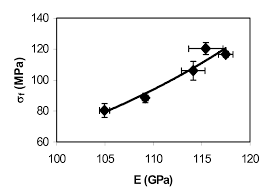## How to Calculate and Solve for Flexural Strength with Relation to Volume Fraction | CeramicsThe image above represents Flexural strength with relation to volume.

To compute for flexural strength with relation to volume, three essential parameters are needed and these parameters are Initial Stress (σo), Constant (n) and Volume fraction porosity (P).

The formula for calculating flexural strength with relation to volume:

σfs = σo exp (-nP)

Where:

σfs = Flexural Strength
σo = Initial Stress
n = Constant
P = Volume Fraction Porosity

Let’s solve an example;
Find the flexural strength when the initial stress is 11, the constant is 8 and the volume fraction porosity is 22.

This implies that;

σo = Initial Stress = 11
n = Constant = 8
P = Volume Fraction Porosity = 22

σfs = σo exp (-nP)
σfr = (11)exp(-(8)(22))
σfr = (11)exp(-176)
σfr = (11)(3.665e-77)
σfr = 4.032e-76

Therefore, the flexural strength is 4.032e-76 Pa.

Calculating the Initial Stress when the Flexural Strength, the Constant and the Volume Fraction Porosity is Given.

σo = σfr / exp (-nP)

Where;

σo = Initial Stress
σfs = Flexural Strength
n = Constant
P = Volume Fraction Porosity

Let’s solve an example;
Find the initial stress when the flexural strength is 20, the constant is 10 and the volume fraction porosity is 8.

This implies that;

σfs = Flexural Strength = 20
n = Constant = 10
P = Volume Fraction Porosity = 8

σo = σfr / exp (-nP)
σo = 20 / exp (-10 x 8)
σo = 20 / exp (-80)
σo = 20 / – 5.54e+34
σo = – 3.61e-34

Therefore, the initial stress is 3.61e-34.